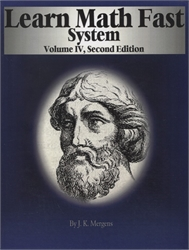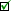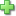# Learn Math Fast System Volume IV

## Basic Geometry

by J. K. Mergens
Consumable Workbook, 148 pages
Current Retail Price: \$38.00
Used Price: \$24.00 (1 in stock)Condition Policy

Publisher Description:

This book teaches basic geometry concepts that are typically taught throughout elementary school.  This is a different order than what Common Core teaches.  We feel that it is too much to ask of a young student to go from long division to fractions to using pi to find the circumference of a circle.  We prefer to teach pre-algebra before asking a student to use formulas such as C = d x pi.  This book shouldn't be skipped unless your student can name the three special triangles, and what's so special about each one, and understands how to use the Pythagorean theorem.  If your student can solve for the length of the hypotenuse in a triangle with sides measuring 6 and 8, then you may skip this book.  After reading this volume, a student will quickly know the answer is 10.

Stand Alone or Supplemental?
Volume 4 covers basic geometry, which typically isn't singled out as a class in public school.  It is usually integrated during basic math.  If your goal is to learn geometry, this is a perfect precursor to volume 7, our high school geometry book.   If you want to prepare your student for algebra, then read volumes 1 - 4 as a stand- alone curriculum.
?

Scope and Sequence (Basic Geometry):
Students will gain knowledge in basic geometric concepts including the difference between straight, parallel and perpendicular lines.
How to identify obtuse, acute, opposite and right angles.
The properties of right, equilateral and isosceles triangles are studied.
The difference between several quadrilaterals and the properties they contain.
Students will learn how to find the area of a triangle, a square and all other polygons.
Pi, radius and diameter are used to find the circumference and area of a circle.
The Pythagorean Theorem is studied and proven to work.
Perimeter of a shape, and volume of a rectangular prism and sphere are learned.
Students will gain knowledge of the Metric System.

Did you find this review helpful?
Series Description
Related Categories
Recommended for...
Customer ReviewsClick here to write a review